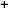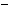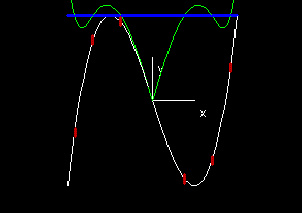Labware - MA35 Multivariable Calculus - Single Variable Calculus
 MA35 Labs 1 » Single Variable Calculus Contents1.3 More Derivatives 1.3.1 Second Derivatives 1.3.3 Derivative Test 1.3.4 Taylor Series 1.3.5 Constrained Maxima & Minima 1.4 Integration Search

Constrained Maxima & Minima

Text

Suppose we want to find the maximum and minimum values of some function f(x) that satisfy the condition that some function g(x) is equal to c.

If g(x) is only equal to c for a finite number of x values (which is true except for a few special cases, such as g(x) being a constant function equal to c), then the solution is simply to see which of these values of x yields the maximum and minimum values of f(x).For functions of more than one variable, it is important to consider the requirement that the gradients of the functions f and g be parallel. For functions of one variable, however, this condition is easily satisfied. The gradient vector in single variable calculus is simply (f' (x)) , so as long as the gradients of f(x) and g(x) are nonzero, they will point directly to the right or directly to the left, making them parallel.Demos

 Constrained Maxima & MinimaThis demo displays all the points on the graph of some function f(x) (shown in white) that satisfy the side condition that some function g(x) (in green) equals some constant c (line y = c in blue). These points are displayed as red tick marks. The constrained maxima here are the points with the highest and lowest values of f(x).Exercises

• 1. Find the constrained maxima and minima in each of the following situations:
• f(x) = x, g(x) = 2x, c = 1
• f(x) = x, g(x) = x3 - x, c = 0
• f(x) = sin(x), g(x) = x2, c = π2/16
• 2. The demo begins with the example g(x) = √(x2 + f(x)2). What is the geometrical significance of g(x)?